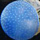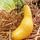## Social Question# Would this be worth showing to a math class?

Asked by LostInParadise (29150) June 9th, 2021

After learning about inverse functions and quadratic equations, I thought students might enjoy seeing a connection between the two.

Suppose we are given the function f(x)= -x**2 + 6x + 10, and we want to find the axis of symmetry. Instead of completing the square we rewrite the function as f(x) = -x(6-x) + 10.

What do we get for f(6 – x)? We notice that 6-x is self-inverse and that f contains terms for both x and 6-x . For f(6-x), x gets mapped to 6 -x and 6-x gets mapped to x. f(6-x)= -(6-x)x + 10, which is the same as f(x), so f(6-x)=f(x). For every point (x,y) on the curve there will be a point (6-x,y) on the curve. The midpoint of (x,y) and (6-x,y) is (3,y), so the line x=3 is a line of symmetry.

This technique can be extended to all quadratic expressions and can be used for other types of functions, like f(x)=arctan(x)*arctan(6-x), which will also have a line of symmetry for x=3.

Observing members: 0Composing members: 0That equation should have been f(x)=x**2–6x+10

LostInParadise (29150)“Great Answer” (1) Flag as…I just blacked out reading this, be nice to the kids. Haha!

KNOWITALL (26285)“Great Answer” (4) Flag as…Let me try another way. We have f(x) = -x(6-x)+10

Take any two numbers that add to 6, like 2 and 4.
x(6-x) in the case of x=2 gives 2×4=8, and in the case of x = 4 we get 4×2=8, the same value. For both x=2 and x=4, we get f(x)=-4×2+10 = -8+10 = 2, so we know that the graph of f(x) has the two points (2,2) and (4,2). The midpoint is (3,2).

Similarly, for any two numbers a and b that add to 6, we will get x(6-x) = ab and f(a)=f(b) = -ab+10 = y for some number y. That means that (a,y) and (b,y) are on the graph and the midpoint is (a+b/2,y) = (3,y), because a+b=6.

We keep getting midpoints of the form (3,y), so the line x=3 must cut the curve in two and is the axis of symmetry.

LostInParadise (29150)“Great Answer” (0) Flag as…You lost me at the word math. All of this sounds like Klingon, translated through Russian, and then through the African clicking language to me.

Kardamom (32198)“Great Answer” (4) Flag as…